# The curve Function

One of the many handy, and perhaps underappreciated, functions in R is curve. It is a neat little function that provides mathematical plotting, e.g., to plot functions. This tutorial shows some basic functionality.

The curve function takes, as its first argument, an R expression. That expression should be a mathematical function in terms of x. For example, if we wanted to plot the line y=x, we would simply type:

curve((x))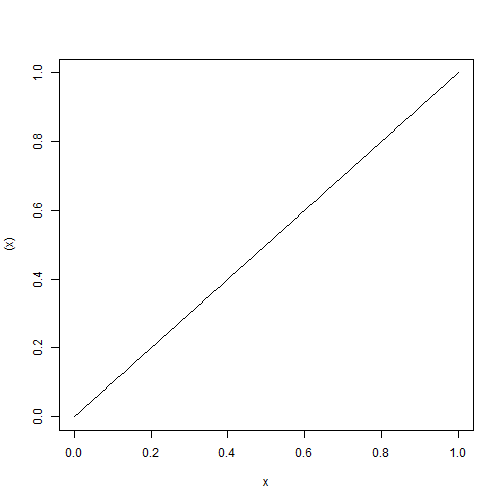Note: We have to type (x) rather than just x.

We can also specify an add parameter to indicate whether to draw the curve on a new plotting device or add to a previous plot. For example, if we wanted to overlay the function y=x^2 on top of y=x we could type:

curve((x))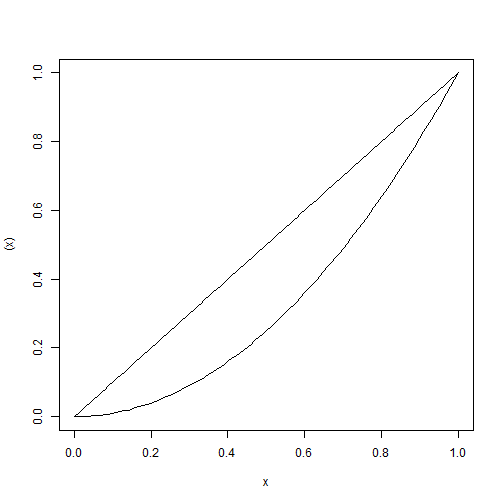We aren't restricted to using curve by itself either. We could plot some data and then use curve to draw a y=x line on top of it:

set.seed(1)
x <- rnorm(100)
y <- x + rnorm(100)
plot(y ~ x)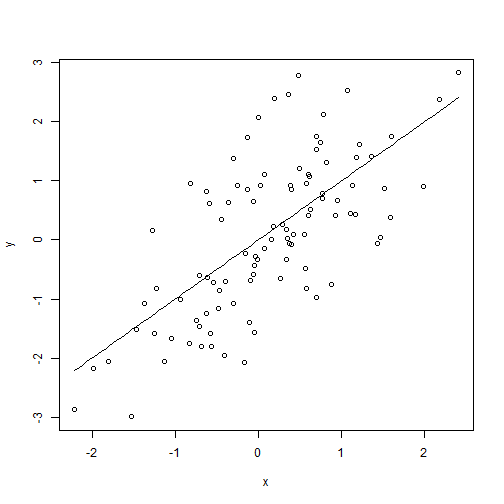And, like all other plotting functions, curve accepts graphical parameters. So we could redraw our previous graph with gray points and a thick red curve:

plot(y ~ x, col = "gray", pch = 15)
curve((x), add = TRUE, col = "red", lwd = 2)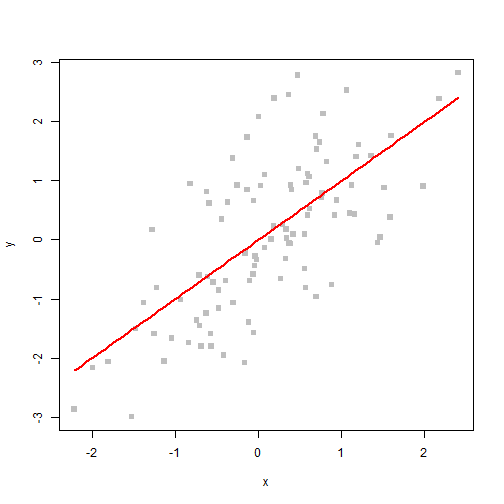We could also call these in the opposite order (replacing plot with points):

curve((x), col = "red", lwd = 2)
points(y ~ x, col = "gray", pch = 15)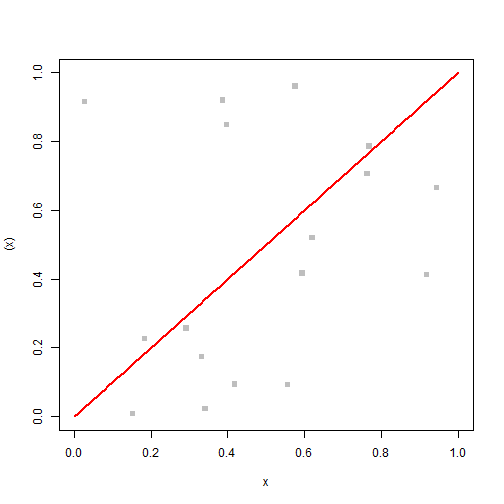Note: The plots are different because calling curve without xlim and ylim plots means that R doesn't know that we're going to add data outside the plotting region when we call points.

We can also use curve (as we would line or points) to draw points rather than a line:

curve(x^2, type = "p")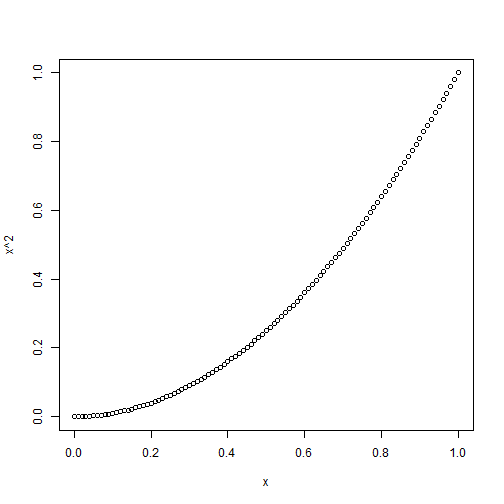We can also specify to and from arguments to determine over what range the curve will be drawn. These are independent of xlim and ylim. So we could draw a curve over a small range on a much larger plotting region:

curve(x^3, from = -2, to = 2, xlim = c(-5, 5), ylim = c(-9, 9))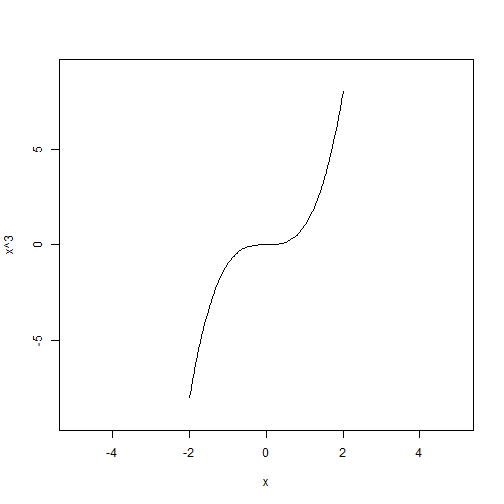Because curve accepts any R expression as its first argument (as long as that expression resolves to a mathematical function of x), we can overlay all kinds of different curves:

curve((x), from = -2, to = 2, lwd = 2)
curve(0 * x, add = TRUE, col = "blue")
curve(0 * x + 1.5, add = TRUE, col = "green")
curve(x^3, add = TRUE, col = "red")
curve(-3 * (x + 2), add = TRUE, col = "orange")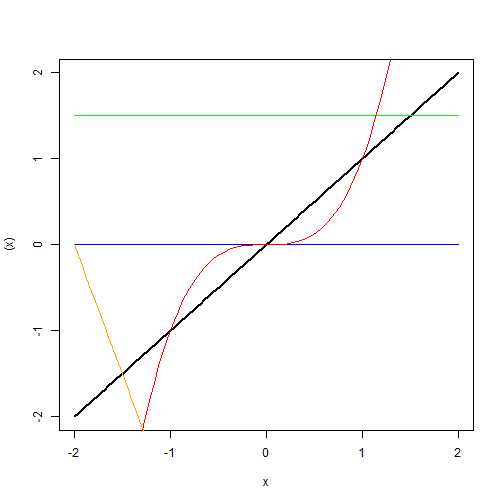These are some relatively basic examples, but they highlight the utility of curve when we simply want to plot a function, it is much easier than generating data vectors that correspond to a function simply for the purposes of plotting.